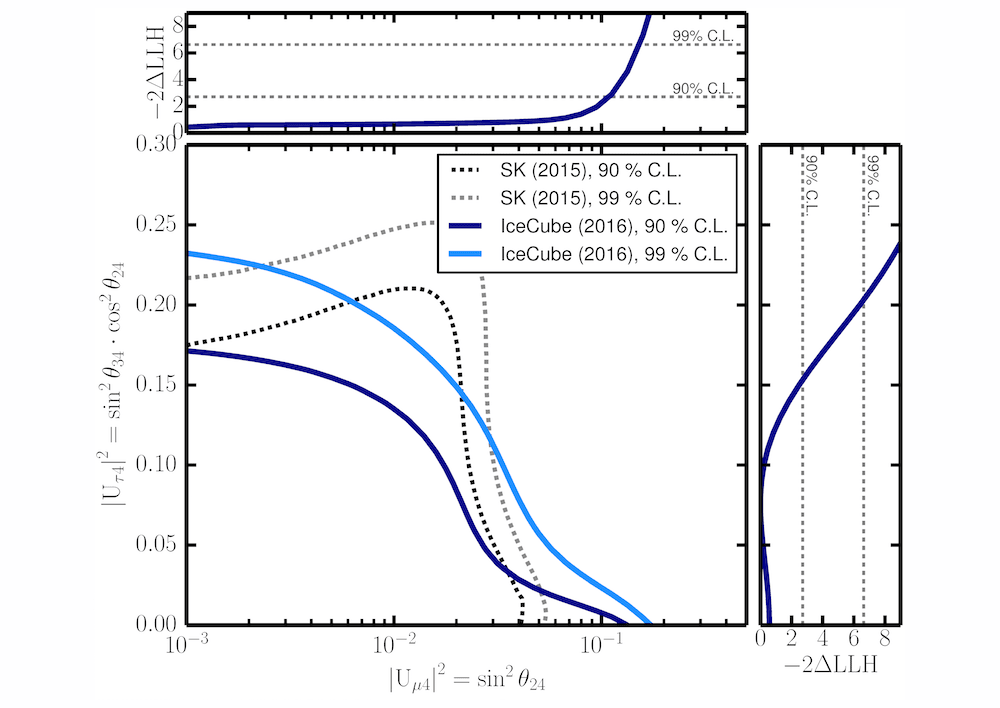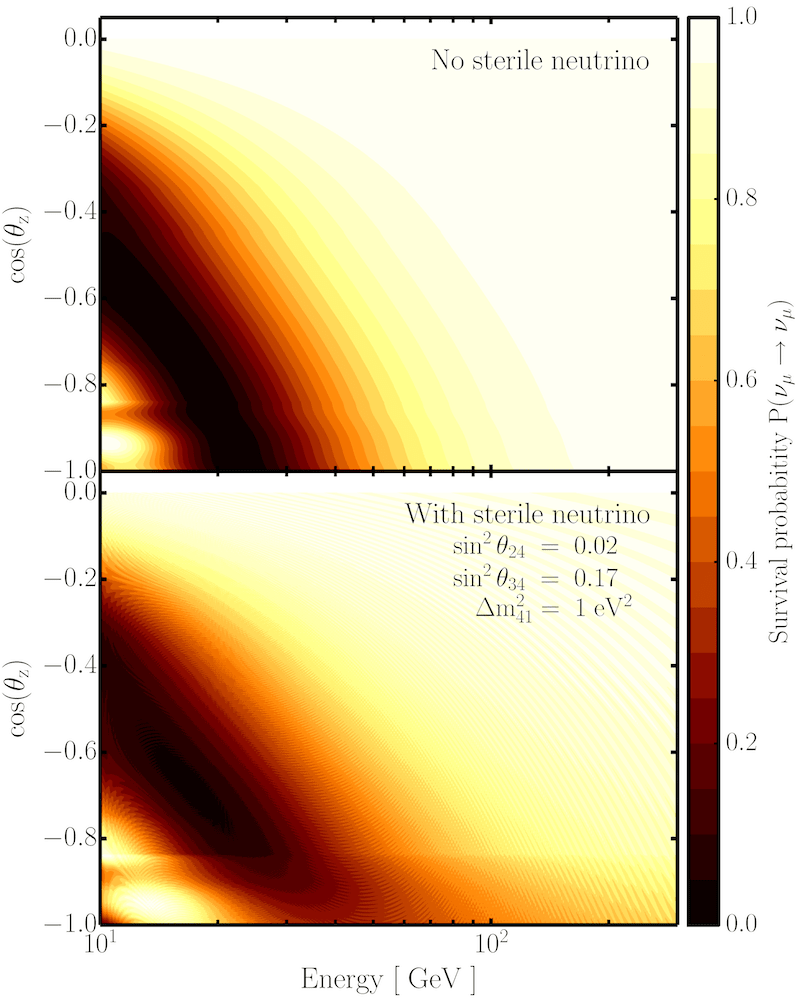# Searching for sterile neutrinos with DeepCore

Last August, IceCube published a first search of sterile neutrinos using high-energy atmospheric neutrinos with energies above 320 GeV. That study came up blank and added more than serious doubt to the existence of light sterile neutrinos.

However, proving the nonexistence of a particle sometimes turns out to be more difficult than proving its existence. The IceCube Collaboration has continued the hunt for sterile neutrinos with a search using lower energy atmospheric neutrinos. This new search looked into three years of IceCube data and again has not found any hint of a light sterile neutrino. IceCube has obtained new exclusion limits for the sterile neutrino oscillation parameters, with a new world-best limit on the |Uτ4|2 mixing matrix element. These results have just been submitted to Physical Review D.The results of the likelihood scan performed in the analysis. The solid lines in the larger panel show the exclusion limits set in this study at 90% (dark blue) and 99% C.L. (light blue). The dashed lines show the exclusion from the Super-Kamiokande experiment. The solid lines on top and right axes show the projection of the likelihood on the mixing matrix elements. Credit: IceCube Collaboration

The existence of a sterile neutrino has been postulated on both a theoretical and an experimental basis. Adding a sterile neutrino to the standard three-neutrino paradigm introduces changes in the oscillation patterns between the three active neutrino states, which is the only way to prove the existence of sterile neutrinos since they do not interact with matter, not even with the weak force.

IceCube can measure neutrino oscillations with neutrinos produced in the interaction of cosmic rays with the atmosphere. Measuring the muon neutrino flux from the Northern Hemisphere, i.e., after those neutrinos have sailed through Earth, we can observe the disappearance of muon neutrinos due to their oscillations to electron and tau neutrinos, and to sterile neutrinos, if they exist.The muon neutrino survival probability for (top) the standard three neutrino oscillations and (bottom) “3+1” sterile neutrino model as a function of true muon neutrino energy and the cosine of the true neutrino zenith angle. Credit: IceCube Collaboration

Neutrino oscillations show a strong disappearance pattern at energies between 10 and 100 GeV. And, if we add one light sterile neutrino, which yields the so-called “3+1 model,” this oscillation pattern shows significant variations. IceCube, thanks to its denser DeepCore subdetector, can detect neutrinos down to energies of approximately 10 GeV and, thus, can probe for the existence of a fourth type of neutrino using neutrino oscillations.

In this study, researchers have searched for a light sterile neutrino using muon neutrino tracks, which were selected from upgoing tracks with an interaction vertex contained in the DeepCore volume. Over 5,000 of these events were found in data taken by the Antarctic neutrino detector between May 2011 and May 2014.

Sterile neutrinos can modify the oscillation pattern through changes in the vacuum oscillations into the sterile neutrino state or the interaction of neutrinos with matter. The first are just due to the total distance travelled from the source to the detector and have a period much smaller than the energy and directional resolution of IceCube’s DeepCore. The second, usually referred to as matter effects, depends on the amount of matter that the neutrinos go through. These matter effects will be stronger when neutrinos reach IceCube after crossing Earth’s core, and they should be especially visible at energies between 20 and 30 GeV.

“Sterile neutrinos just sail the Earth without feeling any of these matter effects,” explains Summer Blot, one of the corresponding authors of this paper and a postdoctoral researcher at DESY. “The presence of sterile neutrinos that oscillate with the standard atmospheric neutrinos would then result in a reduced disappearance rate for neutrinos crossing the Earth’s core with energies around 25 GeV,” adds Blot.

However, the observed muon neutrino flux in IceCube, measured for energies from 10 to 60 GeV, was consistent with the standard three-neutrino hypothesis, adding another null result to the international search for sterile neutrinos. IceCube researchers have used this study to derive new limits on the mixing matrix elements, which resulted in

$$|U_{\mu4}|^2 \lt 0.12$$

and

$$|U_{\tau4}|^{4} \lt 0.15$$

for

$$\Delta m^{2}_{41} \gt 0.3 eV^2$$

The limit on the |Uτ4|2 parameter improves previous world-best limits by approximately 20%.

“IceCube has a unique sample of high quality atmospheric neutrino data. This gives us an ability to set stringent limits on the least constrained sterile neutrino mixing with tau neutrinos,” points out Andrii Terliuk, also a corresponding author of this work and a graduate student at DESY.

+ info “Search for sterile neutrino mixing using three years of IceCube DeepCore data,” The IceCube Collaboration: M. G. Aartsen et al. Physical Review D95 (2017) 11, iopscience.iop.org arxiv.org/abs/1702.05160